Main Content

## Transform Time Series Using Econometric Modeler App

The Econometric Modeler app enables you to transform time series data based on deterministic or stochastic trends you see in plots or hypothesis test conclusions. Available transformations in the app are log, seasonal and nonseasonal difference, and linear detrend. These examples show how to apply each transformation to time series data.

### Apply Log Transformation to Data

This example shows how to stabilize a time series, whose variability grows with the level of the series, by applying the log transformation. The data set `Data_Airline.mat` contains monthly counts of airline passengers.

At the command line, load the `Data_Airline.mat` data set.

`load Data_Airline`

At the command line, open the Econometric Modeler app.

`econometricModeler`

Alternatively, open the app from the apps gallery (see Econometric Modeler).

Import `DataTable` into the app:

1. On the Econometric Modeler tab, in the Import section, click.

2. In the Import Data dialog box, in the Import? column, select the check box for the `DataTable` variable.

3. Click .

The variable `PSSG` appears in the Time Series pane, and its time series plot is in the Time Series Plot(PSSG) figure window.

Fit a SARIMA(0,1,1)×(0,1,1)12 model to the data in levels:

1. On the Econometric Modeler tab, in the Models section, click the arrow to display the model gallery.

2. In the models gallery, in the ARMA/ARIMA Models section, click SARIMA.

3. In the SARIMA Model Parameters dialog box, on the Lag Order tab:

• Nonseasonal section

1. Set Degrees of Integration to `1`.

2. Set Moving Average Order to `1`.

3. Clear the Include Constant Term check box.

• Seasonal section

1. Set Period to `12` to indicate monthly data.

2. Set Moving Average Order to `1`.

3. Select the Include Seasonal Difference check box.4. Click .

The model variable `SARIMA_PSSG` appears in the Models pane, its value appears in the Preview pane, and its estimation summary appears in the Model Summary(SARIMA_PSSG) document.The spread of the residuals increases with the level of the data, which is indicative of heteroscedasticity.

Apply the log transform to `PSSG`:

1. In the Time Series pane, select `PSSG`.

2. On the Econometric Modeler tab, in the Transforms section, click .

The transformed variable `PSSGLog` appears in the Time Series pane, and its time series plot appears in the Time Series Plot(PSSGLog) figure window.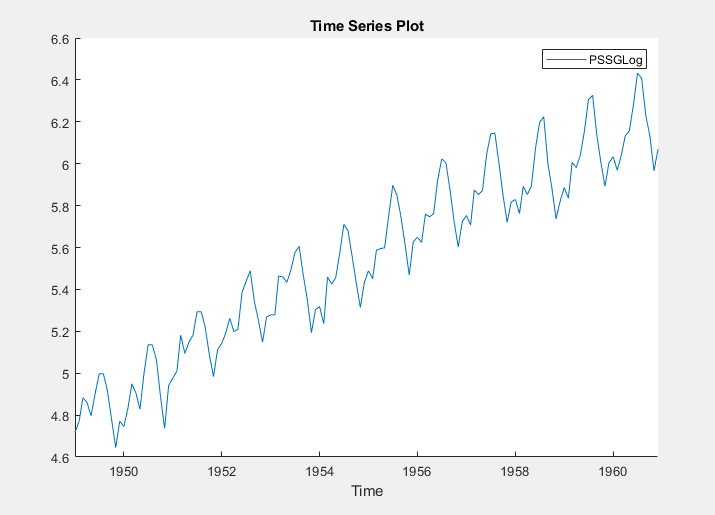The exponential growth appears removed from the series.

With `PSSGLog` selected in the Time Series pane, fit the SARIMA(0,1,1)×(0,1,1)12 model to the logged series using the same dialog box settings that you used for `PSSG`. The estimation summary appears in the Model Summary(SARIMA_PSSGLog) document.The spread of the residuals does not appear to change systematically with the levels of the data.

### Stabilize Time Series Using Nonseasonal Differencing

This example shows how to stabilize a time series by applying multiple nonseasonal difference operations. The data set, which is stored in `Data_USEconModel.mat`, contains the US gross domestic product (GDP) measured quarterly, among other series.

At the command line, load the `Data_USEconModel.mat` data set.

`load Data_USEconModel`

At the command line, open the Econometric Modeler app.

`econometricModeler`

Alternatively, open the app from the apps gallery (see Econometric Modeler).

Import `DataTable` into the app:

1. On the Econometric Modeler tab, in the Import section, click.

2. In the Import Data dialog box, in the Import? column, select the check box for the `DataTable` variable.

3. Click .

The variables, including `GDP`, appear in the Time Series pane, and a time series plot of all the series appears in the Time Series Plot(COE) figure window.

In the Time Series pane, double-click `GDP`. A time series plot of `GDP` appears in the Time Series Plot(GDP) figure window.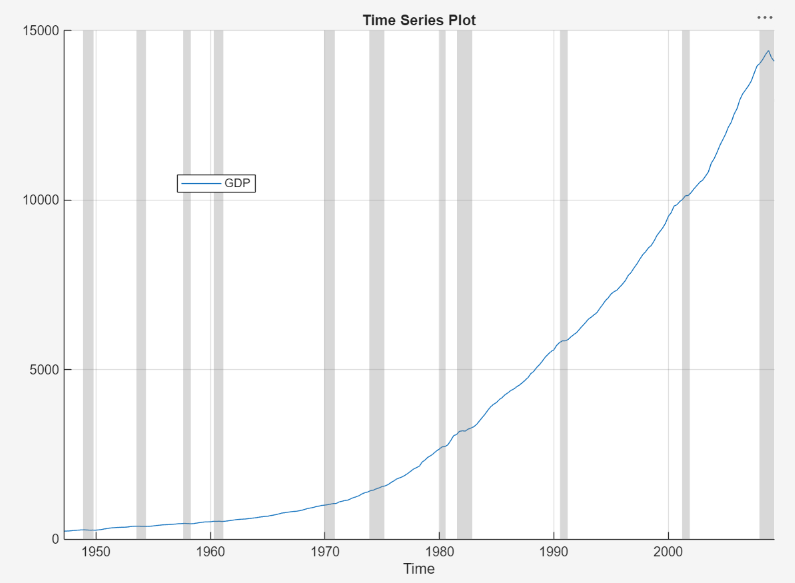The series appears to grow without bound.

Apply the first difference to `GDP`. On the Econometric Modeler tab, in the Transforms section, click .

In the Time Series pane, a variable representing the differenced GDP (`GDPDiff`) appears. A time series plot of the differenced GDP appears in the Time Series Plot(GDPDiff) figure window.The differenced GDP series appears to grow without bound after 1970.

Apply the second difference to the GDP by differencing the differenced GDP. With `GDPDiff` selected in the Time Series pane, on the Econometric Modeler tab, in the Transforms section, click .

In the Time Series pane, a variable representing the transformed differenced GDP (`GDPDiffDiff`) appears. A time series plot of the differenced GDP appears in the Time Series Plot(GDPDiffDiff) figure window.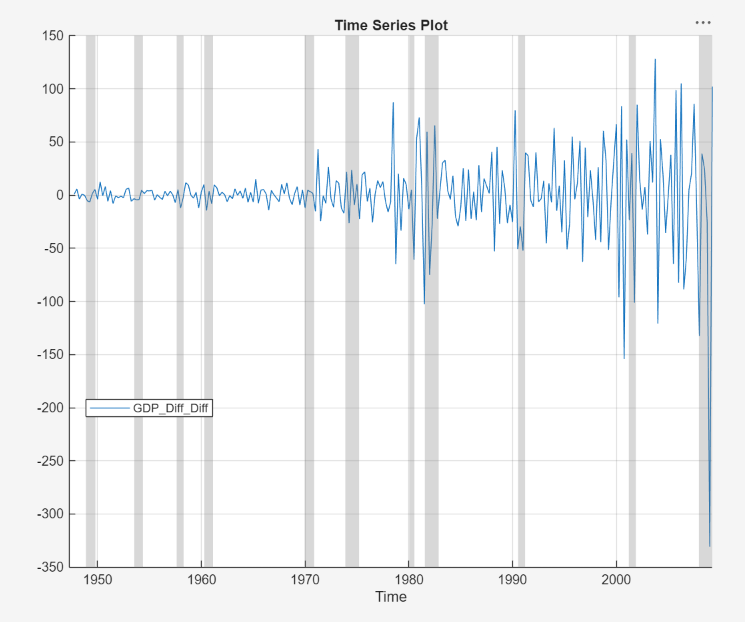The transformed differenced GDP series appears stationary, although heteroscedastic.

### Convert Prices to Returns

This example shows how to convert multiple series of prices to returns. The data set, which is stored in `Data_USEconModel.mat`, contains the US GDP and personal consumption expenditures measured quarterly, among other series.

At the command line, load the `Data_USEconModel.mat` data set.

`load Data_USEconModel`

At the command line, open the Econometric Modeler app.

`econometricModeler`

Alternatively, open the app from the apps gallery (see Econometric Modeler).

Import `DataTable` into the app:

1. On the Econometric Modeler tab, in the Import section, click.

2. In the Import Data dialog box, in the Import? column, select the check box for the `DataTable` variable.

3. Click .

`GDP` and `PCEC`, among other series, appear in the Time Series pane, and a time series plot containing all series appears in the figure window.

In the Time Series pane, click `GDP`, then press Ctrl and click `PCEC`. Both series are selected.

Click the Plots tab, then click . A time series plot of `GDP` and `PCEC` appears in the Time Series Plot(GDP) figure window.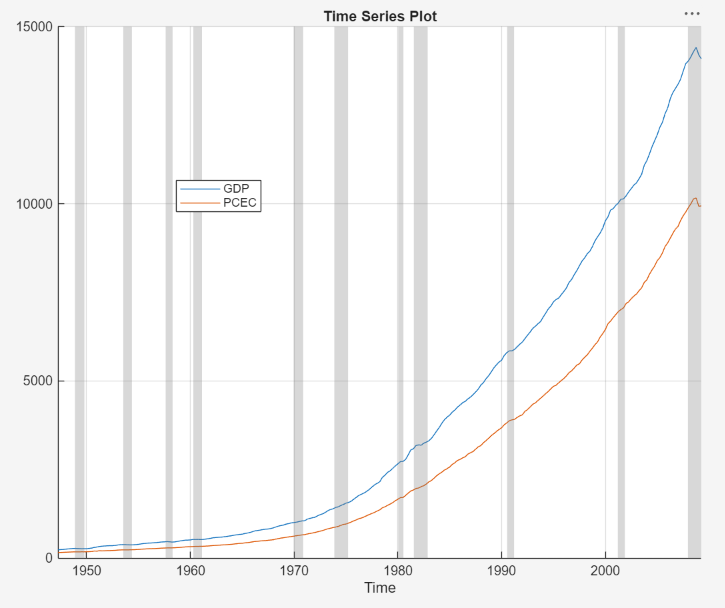Both series, as prices, appear to grow without bound.

Convert the GDP and personal consumption expenditure prices to returns:

1. Click the Econometric Modeler tab. Ensure that `GDP` and `PCEC` are selected in the Time Series pane.

2. In the Transforms section, click .

The Time Series pane displays variables representing the logged GDP series (`GDPLog`) and the logged personal consumption expenditure series (`PCECLog`).

3. With `GDPLog` and `PCECLog` selected in the Time Series pane, in the Transforms section, click .

The Time Series pane displays variables representing the GDP returns (`GDPLogDiff`) and personal consumption expenditure returns (`PCECLogDiff`). A time series plot of the GDP and personal consumption expenditure returns appears in the Time Series Plot(GDPLogDiff) figure window.

In the Time Series pane, rename the `GDPLogDiff` and `PCECLogDiff` variables. Click `GDPLogDiff` twice to select its name and enter `GDPReturns`. Click `PCECLogDiff` twice to select its name and enter `PCECReturns`.

The app updates the names of all documents associated with both returns.The series of GDP and personal consumption expenditure returns appear stationary, but observations within each series appear serially correlated.

### Remove Seasonal Trend from Time Series Using Seasonal Difference

This example shows how to stabilize a time series exhibiting seasonal integration by applying a seasonal difference. The data set `Data_Airline.mat` contains monthly counts of airline passengers.

At the command line, load the `Data_Airline.mat` data set.

`load Data_Airline`

At the command line, open the Econometric Modeler app.

`econometricModeler`

Alternatively, open the app from the apps gallery (see Econometric Modeler).

Import `DataTable` into the app:

1. On the Econometric Modeler tab, in the Import section, click.

2. In the Import Data dialog box, in the Import? column, select the check box for the `DataTable` variable.

3. Click .

The variable `PSSG` appears in the Time Series pane, and its time series plot appears in the Time Series Plot(PSSG) figure window.

Address the seasonal trend by applying the 12th order seasonal difference. On the Econometric Modeler tab, in the Transforms section, set Seasonal to `12`. Then, click .

The transformed variable `PSSGSeasonalDiff` appears in the Time Series pane, and its time series plot appears in the Time Series Plot(PSSGSeasonalDiff) figure window.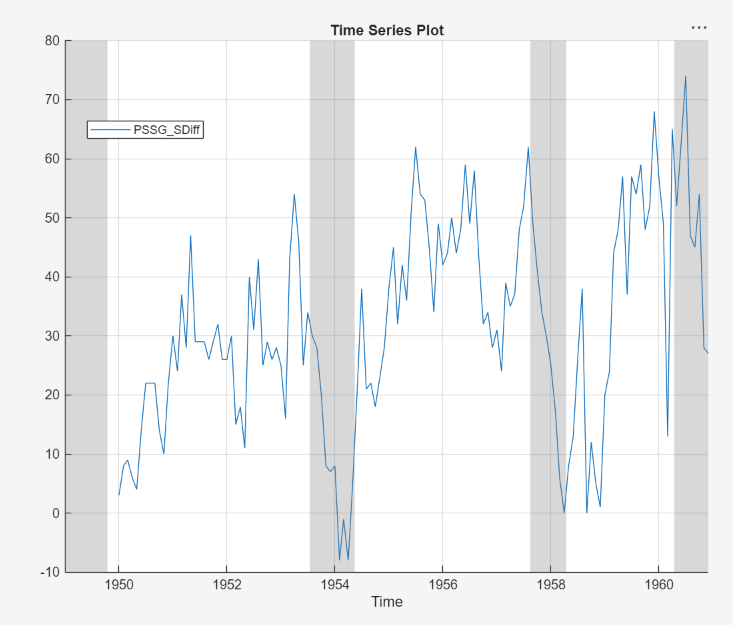The transformed series appears to have a nonseasonal trend.

Address the nonseasonal trend by applying the first difference. With `PSSGSeasonalDiff` selected in the Time Series pane, on the Econometric Modeler tab, in the Transforms section, click .

The transformed variable `PSSGSeasonalDiffDiff` appears in the Time Series pane, and its time series plot appears in the Time Series Plot(PSSGSeasonalDiffDiff) figure window.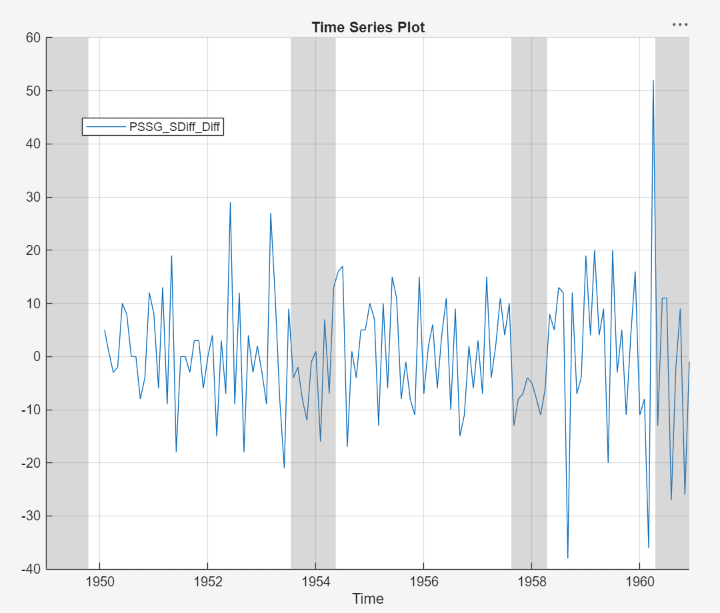The transformed series appears stationary, but observations appear serially correlated.

In the Time Series pane, rename the `PSSGSeasonalDiffDiff` variable by clicking it twice to select its name and entering `PSSGStable`.

The app updates the names of all documents associated with the transformed series.

### Remove Deterministic Trend from Time Series

This example shows how to remove a least-squares-derived deterministic trend from a nonstationary time series. The data set `Data_Airline.mat` contains monthly counts of airline passengers.

At the command line, load the `Data_Airline.mat` data set.

`load Data_Airline`

At the command line, open the Econometric Modeler app.

`econometricModeler`

Alternatively, open the app from the apps gallery (see Econometric Modeler).

Import `DataTable` into the app:

1. On the Econometric Modeler tab, in the Import section, click.

2. In the Import Data dialog box, in the Import? column, select the check box for the `DataTable` variable.

3. Click .

The variable `PSSG` appears in the Time Series pane, and its time series plot appears in the Time Series Plot(PSSG) figure window.

Apply the log transformation to the series. On the Econometric Modeler tab, in the Transforms section, click Log.

The transformed variable `PSSGLog` appears in the Time Series pane, and its time series plot appears in the Time Series Plot(PSSGLog) figure window.

Identify the deterministic trend by using least squares. Then, detrend the series by removing the identified deterministic trend. On the Econometric Modeler tab, in the Transforms section, click Detrend.

The transformed variable `PSSGLogDetrend` appears in the Time Series pane, and its time series plot appears in the Time Series Plot(PSSGLogDetrend) figure window.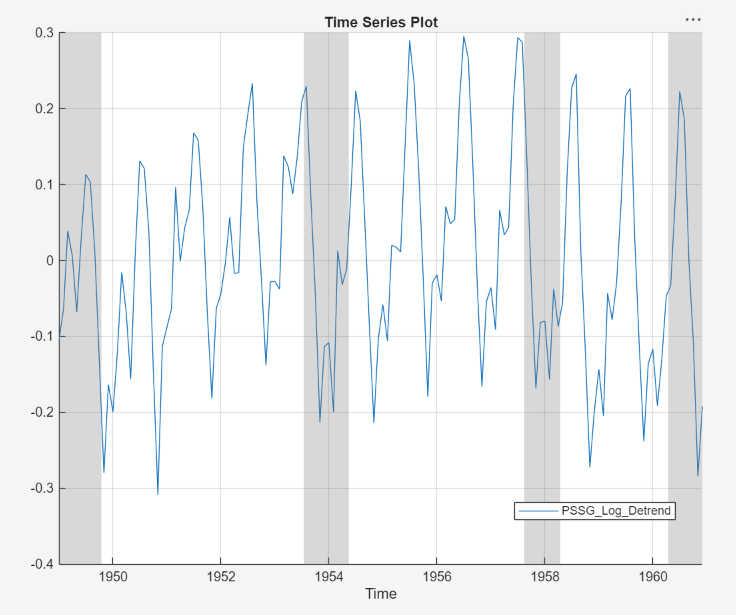`PSSGLogDetrend` does not appear to have a deterministic trend, although it has a marked cyclic trend.

Download ebook